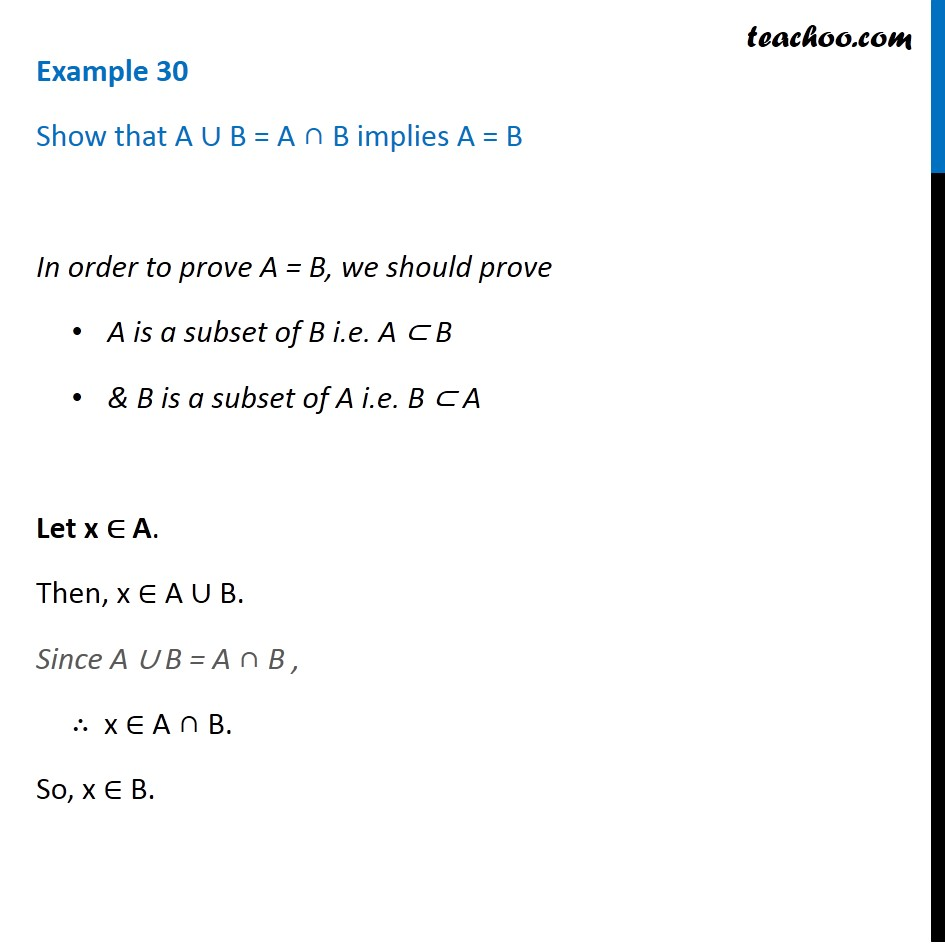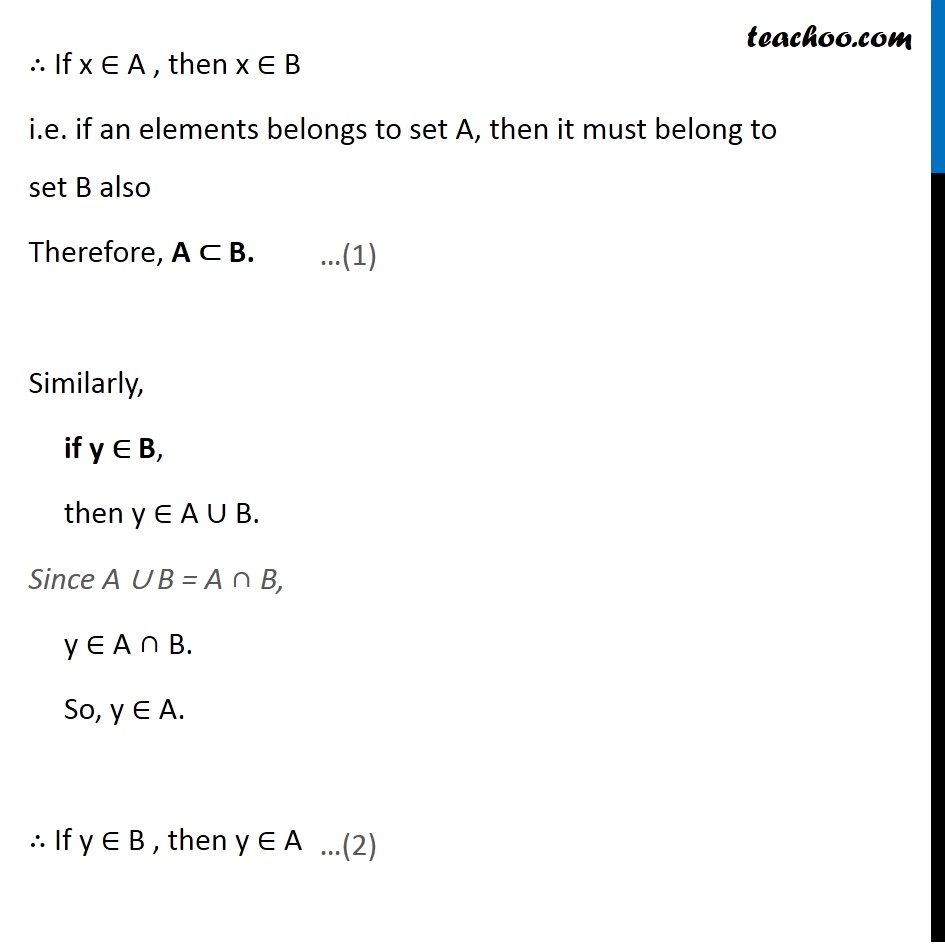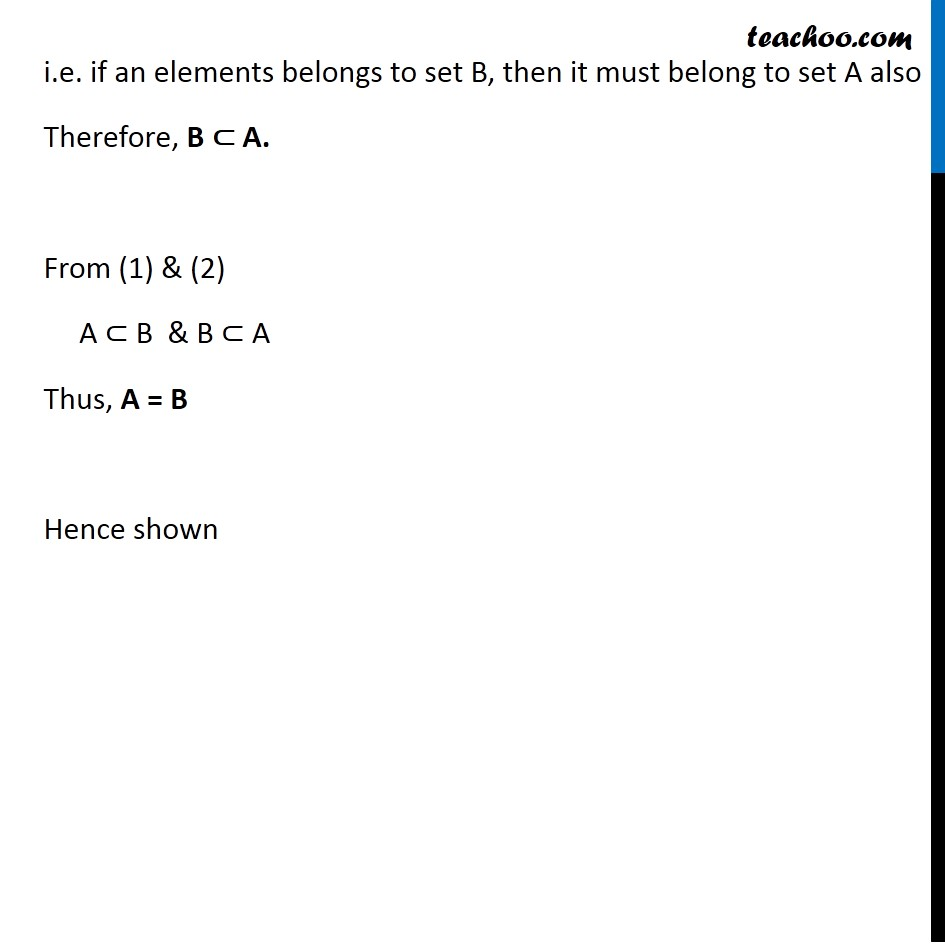1. Chapter 1 Class 11 Sets
2. Concept wise
3. Proof - where properties of sets cant be applied,using element

Transcript

Example 30 Show that A ∪ B = A ∩ B implies A = B In order to prove A = B, we should prove A is a subset of B i.e. A ⊂ B & B is a subset of A i.e. B ⊂ A Let x ∈ A. Then, x ∈ A ∪ B. Since A ∪ B = A ∩ B , ∴ x ∈ A ∩ B. So, x ∈ B. ∴ If x ∈ A , then x ∈ B i.e. if an elements belongs to set A, then it must belong to set B also Therefore, A ⊂ B. Similarly, if y ∈ B, then y ∈ A ∪ B. Since A ∪ B = A ∩ B, y ∈ A ∩ B. So, y ∈ A. ∴ If y ∈ B , then y ∈ A …(1) i.e. if an elements belongs to set B, then it must belong to set A also Therefore, B ⊂ A. From (1) & (2) A ⊂ B & B ⊂ A Thus, A = B Hence shown

Proof - where properties of sets cant be applied,using element